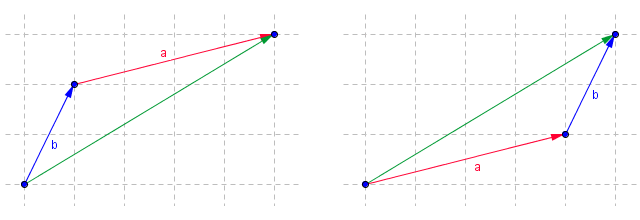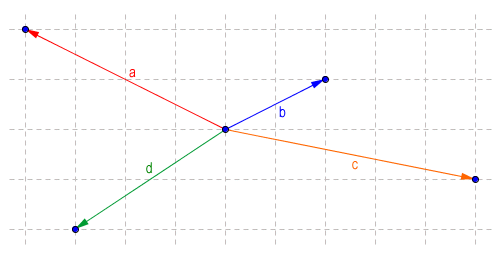Adding two (or more) vectors together always results in another vector, called the resultant. The vectors being added together are known as the components of the resultant vector. Unless the component vectors are acting in the same direction to start with however, the direction of the resultant will be different to that of either of its components. Furthermore, the absolute magnitude of the resultant (i.e. its magnitude ignoring sign) will usually be smaller than the sum of the absolute magnitudes of its component vectors (unless the component vectors are all acting in the same direction). You probably already know that any vector in a two-dimensional plane can be described in terms of its x and y components, and that these components are themselves vectors. To visualise what this means, imagine yourself walking three kilometres due east, then four kilometres due north. You have walked seven kilometres in all, but how far are you from the point where you started, in a straight line?How far are you from the pont of origin?

Looking at the above diagram, the answer (five kilometres) can be found easily enough by applying Pythagoras' theorem. Each leg of your journey (the three kilometres east, and the four kilometres north) is a separate vector. The two vectors form the legs of a right-angled triangle, for which the line segment connecting the point at which you started and the the point where you ended up (i.e. the resultant) becomes the hypotenuse. Here is the calculation:

distance from origin = √32 + 42 = 5

So, although you have actually walked seven kilometres, you are really only five kilometers from your point of origin. This is of course perfectly logical, since the shortest distance between two points is always a straight line. We can use this kind of visual representation to find a resultant for the addition of any set of vectors. The illustration below shows two free vectors, a and b.Free vectors a and b

If we want to visualise what happens when we add these vectors together, we can move one of the vectors (without changing its direction) so that its tail is touching the tip of the arrow head belonging to the other vector. It doesn't really matter which vector we choose to move - the result will be the same (as for the addition of scalar values, the addition of vectors is both commutative and associative). To show that this is the case, the illustration below shows both possible scenarios. In each case, the resultant is shown as a green arrow.A graphic representation of vector addition

We can do something very similar to represent subtracting one vector from another. If we want to subtract vector b from vector a, for example, we simply reverse the direction of vector b to create a new vector called -b, and follow the same procedure as previously, i.e. move vector -b so that its tail is touching the tip of the arrow head belonging to vector a. Note however that, just as for scalar values, subtraction is neither commutative nor associative. We will not get the same result if we instead subtract vector a from vector b. To demonstrate this, both scenarios are illustrated below. Note that although the magnitude of the resultant is the same in both cases, its direction when vector a is subtracted from vector b is completely opposite to the direction of the vector that results from subtracting vector b from vector a.Vector subtraction is non-commutative and non-associative

One point to note here is that if we wish to subtract one vector from another, we can essentially just reverse the direction of the vector to be subtracted, and then add the two vectors together. The other point to note is that the resultant goes from the chosen point of origin to the end point directly. The component vectors (i.e. the vectors we are adding together) create an indirect path from the same point of origin to the same end point. Logically, therefore, the sum of the x components of the two vectors to be added must equal the x component of the resultant, and the sum of the y components of the vectors must equal the y component of the resultant. This means that we can express a vector addition using numbers, rather than having to draw a diagram each time we want to add vectors together. Let's carry out our original vector addition again, this time using matrix addition to find the x and y components of the resultant:

 a + b = 4 + 1 = 5 1 2 3

If we want to subtract vector b from vector a, we simply negate the x and y component values for vector b as shown here:

 a - b = 4 + -1 = 3 1 -2 -1

Similarly, we can subtract vector a from vector b, by negating the x and y component values for vector a:

 b - a = 1 + -4 = -3 2 -1 1

If you check the results obtained here with the illustrations above, you should be able to see that they are consistent with the results we obtained using a visual representation of vector addition. While you're at it you can also satisfy yourself that the x and y components given for vectors a and b are correct (you can do this by counting the number of squares, in each of the horizontal and vertical directions, between the tail of the arrow representing the vector and its tip). For the sake of convenience, we have used integer values for the x and y components of all vectors. In real life, a component value will often have a fractional part, but the principle is the same. Adding vectors is relatively easy, providing you know the values of the x and y components of each vector.

Although we have so far only added two vectors together, the process works equally well for any number of vectors. Consider the scenario illustrated below, which shows four free vectors. What do you think will be the result of adding them all together?What will be the result of adding these vectors?

We could of course find the answer using the visual method we used previously, but let's first try the vector addition using a numerical method. We need to know the x and y components for each vector, which we can find by counting squares. Here is a list of the vectors, with their x and y components in row vector form:

a = (-4, 2)

b = (2, 1)

c = (5, -1)

d = (-3, -2)

Now we have the information we need, we can put everything into matrix form:

 a + b + c + d = -4 + 2 + 5 + -3 = 0 2 1 -1 -2 0

According to the above calculation, adding vectors a, b, c and d produces a resultant for which both the x component and the y component are zero. The only conclusion we can draw from this is that if we travel along each of the vectors in turn, in whatever order we choose (remembering that the order is unimportant when adding vectors), then we will always end up back at the point where we started. This is indeed the case, as the figure below illustrates. You can play around with different orderings of these vectors, but they will always lead back to the same point. Note that a vector for which both the x and y components are zero is called the zero vector (or null vector).The resultant of adding these vectors is the zero vector (0, 0)

Vector addition works the same way for three dimensions (or any number of dimensions, for that matter). The diagram below shows three vectors, a, b and c. The resultant created by adding these vectors together is also shown. Note that the relative direction of the axes has been chosen somewhat arbitrarily. When working in a computer graphics environment, the convention is for the horizontal axis to be the x-axis and the vertical axis to be the y-axis. The z-axis is then the axis that is perpendicular to both the x and y axes. Positive z values extend out of the screen (towards the user), and negative z values extend into the screen (away from the user).The resultant is the sum of vectors a, b and c

For those interested in such things, the above diagram was produced using GeoGebra 5.0 Beta, which is at the time of writing still under development. This software is freely available under the GNU General Public License (website: www.geogebra.org). The vector arithmetic is shown below, and is very similar to what we have already seen. The only significant difference is that we have one extra component (the z component) that must be included in our calculation. There is one more thing to note about vector addition - you can only add vectors that have the same number of components. You cannot, for example, add a two dimensional vector to a three dimensional vector.

 a + b + c = -2 + -2 + 3 = -1 6 -5 4 5 2 3 1 6